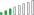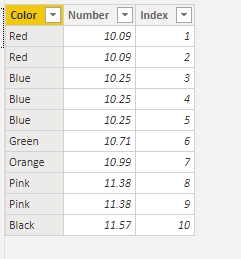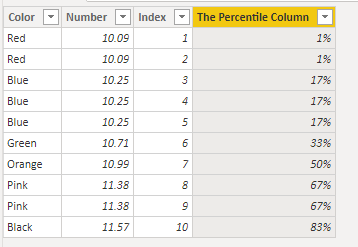cancel
Showing results for
Did you mean:Regular Visitor

## Percentiles based on another column

Hi all,

I would like to be able to specify a percentage, let's say 50% of the distinct values in column A. So in this case (0.5 * 6 distinct colours = 3). And for the output to return a value for which there are that many colours above in column B.

For example:

50% of 6 colours is 3 and therefore the output would be a B value between 10.71 and 10.99 because then there would be Orange, Pink and Blue with a value above. Please see below table for clarity.Really difficult one to explain so I hope that makes sense, any help would be really appreciated. Not even sure if it's at all possible. Have been playing around with the DAX Percentile function however can only get it to return the percentile based on column B which doesn't give me what I need.

1 ACCEPTED SOLUTIONResolver III

I think I see what you are trying to do here. There are a bunch of ways we could go about doing this. Let me know if this helps.

I am assuming you want to treat each row as a different score so I've included an Index column to make each entry have a unique ID. But as you mentioned you want percentile based on the color and not based on the individual unique row scores.First we can make a measure that sums the total numerical value

``````Total Number =
SUM(Data[Number])``````

Next we can make our Percentile calculated column.

``````The Percentile Column =

var TheNumber= [Total Number]

var TheCalc =         CALCULATE(DISTINCTCOUNT(Data[Color]), FILTER(ALL(Data), Data[Number] < TheNumber))
/
CALCULATE(DISTINCTCOUNT(Data[Color]), ALL(Data)) + 0
return

IF(TheCalc = 0, .01, TheCalc)``````

Now our table will look like thisFrom here you should be able to select the filters and create the measures you are looking for.

2 REPLIES 2Resolver III

I think I see what you are trying to do here. There are a bunch of ways we could go about doing this. Let me know if this helps.

I am assuming you want to treat each row as a different score so I've included an Index column to make each entry have a unique ID. But as you mentioned you want percentile based on the color and not based on the individual unique row scores.First we can make a measure that sums the total numerical value

``````Total Number =
SUM(Data[Number])``````

Next we can make our Percentile calculated column.

``````The Percentile Column =

var TheNumber= [Total Number]

var TheCalc =         CALCULATE(DISTINCTCOUNT(Data[Color]), FILTER(ALL(Data), Data[Number] < TheNumber))
/
CALCULATE(DISTINCTCOUNT(Data[Color]), ALL(Data)) + 0
return

IF(TheCalc = 0, .01, TheCalc)``````

Now our table will look like thisFrom here you should be able to select the filters and create the measures you are looking for.Regular Visitor

That seems to have done the job.

Many thanks, really appreciate you taking the time to help me out!